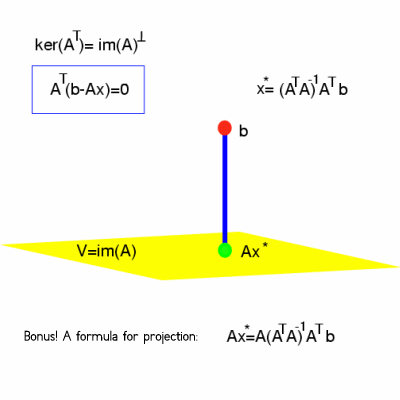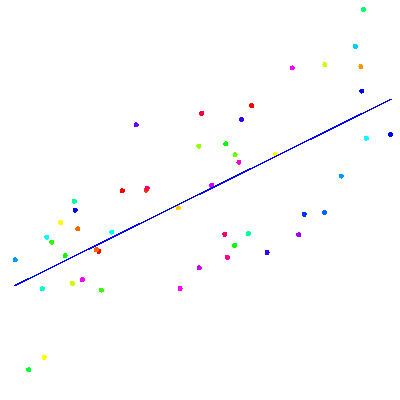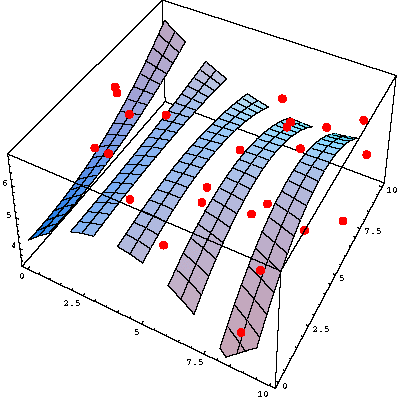Fall 2003

# Mathematics Math21b Fall 2003

## Linear Algebra and Differential Equations

Office: SciCtr 434
Email: knill@math.harvard.edu# Data Fitting

(least square solutions)

## Least Square SolutionYou have seen the method to fit data (x1,y1),..., (xm,ym) by functions of the form f=a1 f1(x)+ ... + an fn(x). The idea was to write a system A a = b of linear equations which tell that all the data can be fitted by the functions f(xi)=yi, then find the least square solution a* = (AT A)-1 AT b to this system. (The least square solution formula was obtained from AT (b-A a) = 0 which paraphrases that the "error" (b-A a) is perpendicular to the image of A. Geometrically this means that A a is the projection of b onto the image of A. )

## RegressionExamples. Linear fitting with a linear function f(x) = a1 x + a2, where n=2:
 ``` a1 x1 + a2 = y2 a1 x1 + a2 = y2 ..... a1 xm + a2 = ym ```
where
 ``` | x1 1 | | x2 2 | A = | ... | | ... | | xm m | ```
and the formula a = (AT A)-1 AT b can be written as a1 = Cov[X,Y]/Var[X], a2 = E[Y]-a1 E[X], where E[X]=x1 + ...+xn is the expectation of the x data, E[Y]=y1 + ...+yn is the expectation of the y data, Var[X]=E[X2]-E[X] is the variance and Cov[X,Y] = E[X Y]-E[X] E[Y] is the covariance of X and Y. The solution line y = a1 x + a2 is called the regression line.

(The same formula can also be obtained using Lagrange extrema by minimizing Var[a1 X + a2 - Y] under the constraint E[a1 X + a2 -Y]=0.)

## Higher dimensionsWe can also fit data (x1,y1,z1), ..., (xm,ym,zm)in three dimensions by functions z=f(x,y) = a1 f1(x,y) + ... + an fn(x,y) or implicitly by surfaces g(x,y,z) = 0 like for example g(x,y,z) = a1x2 + a2 y2 + a3 z2 + a4, in which case one would want to find the best ellipsoid centered at the origin which fits the three dimensional data.

Back to the main page